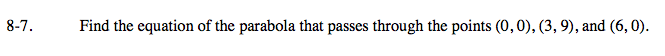### Home > A2C > Chapter 8 > Lesson 8.1.1 > Problem8-7

8-7.There are three ways to approach this problem.

Use the x-intercepts.

Notice that (0, 0) and (6, 0) are x-intercepts.
What are the factors?

The equation is y = ax(x − 6).
Substitute (3, 9) into the equation and solve for a.

Use the vertex.

Notice that since 3 is midway between 0 and 6, (3, 9) is the vertex.
Write the equation in vertex form.

The equation is
y = a(x − 3)² + 9.
Substitute one of the
x-intercepts into the equation and solve for a.

Standard form.

Substitute the three points into the equation
y = ax² + bx + c to make a system of equations in three variables.

Since you know what c is you can substitute it into all of the equations and solve a system of two equations in two variables.